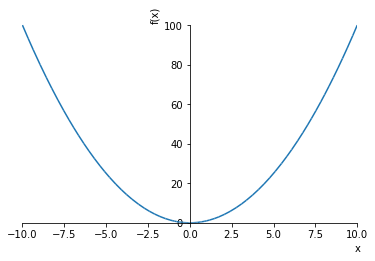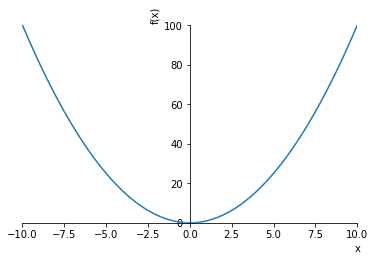# Symbolic mathematics with Sympy¶

Sympy is described as a:

"... Python library for symbolic mathematics."

This means it can be used to:

• Manipulate symbolic expressions;
• Solve symbolic equations;
• Carry out symbolic Calculus;
• Plot symbolic function.

It has other capabilities that we will not go in to in this handbook. But you can read more about it here: http://www.sympy.org/en/index.html

## Manipulating symbolic expressions¶

Before we can start using the library to manipulate expressions, we need to import it.

In :
import sympy as sym


The above imports the library and gives us access to it's commands using the shortand sym which is conventially used.

If we wanted to get Python to check that $x - x = 0$ we would get an error if we did not tell Python what $x$ was.

This is where Sympy comes in, we can tell Python to create $x$ as a symbolic variable:

In :
x = sym.symbols('x')


Now we can calculate $x - x$:

In :
x - x

Out:
0

We can create and manipulate expressions in Sympy. Let us for example verify:

$$(a + b) ^ 2 = a ^ 2 + 2ab + b ^2$$

First, we create the symbolic variables $a, b$:

In :
a, b = sym.symbols('a, b')


Now let's create our expression:

In :
expr = (a + b) ** 2
expr

Out:
(a + b)**2

Note we can get Sympy to use LaTeX so that the output looks nice in a notebook:

In :
sym.init_printing()

In :
expr

Out:
$$\left(a + b\right)^{2}$$

Let us expand our expression:

In :
expr.expand()

Out:
$$a^{2} + 2 a b + b^{2}$$

Note that we can also get Sympy to produce the LaTeX code for future use:

In :
sym.latex(expr.expand())

Out:
'a^{2} + 2 a b + b^{2}'

EXERCISE Use Sympy to verify the following expressions:

• $(a - b) ^ 2 = a ^ 2 - 2 a b + b^2$
• $a ^ 2 - b ^ 2 = (a - b) (a + b)$ (instead of using expand, try factor)

## Solving symbolic equations¶

We can use Sympy to solve symbolic expression. For example let's find the solution in $x$ of the quadratic equation:

$$a x ^ 2 + b x + c = 0$$
In :
# We only really need to define c but doing them all again.
a, b, c, x = sym.symbols('a, b, c, x')


The Sympy command for solving equations is solveset. The first argument is an expression for which the root will be found. The second argument is the value that we are solving for.

In :
sym.solveset(a * x ** 2 + b * x + c, x)

Out:
$$\left\{- \frac{b}{2 a} - \frac{1}{2 a} \sqrt{- 4 a c + b^{2}}, - \frac{b}{2 a} + \frac{1}{2 a} \sqrt{- 4 a c + b^{2}}\right\}$$

EXERCISE Use Sympy to find the solutions to the generic cubic equation:

$$a x ^ 3 + b x ^ 2 + c x + d = 0$$

It is possible to pass more arguments to solveset for example to constrain the solution space. Let us see what the solution of the following is in $\mathbb{R}$:

$$x^2=-1$$
In :
sym.solveset(x ** 2 + 1, x, domain=sym.S.Reals)

Out:
$$\emptyset$$

EXERCISE Use Sympy to find the solutions to the following equations:

• $x ^ 2 == 2$ in $\mathbb{N}$;
• $x ^ 3 + 2 x = 0$ in $\mathbb{R}$.

## Symbolic calculus¶

We can use Sympy to compute limits. Let us calculate:

$$\lim_{x\to 0^+}\frac{1}{x}$$
In :
sym.limit(1/x, x, 0, dir="+")

Out:
$$\infty$$

EXERCISE Compute the following limits:

1. $\lim_{x\to 0^-}\frac{1}{x}$
2. $\lim_{x\to 0}\frac{1}{x^2}$

We can use also Sympy to differentiate and integrate. Let us experiment with differentiating the following expression:

$$x ^ 2 - \cos(x)$$
In :
sym.diff(x ** 2 - sym.cos(x), x)

Out:
$$2 x + \sin{\left (x \right )}$$

Similarly we can integrate:

In :
sym.integrate(x ** 2 - sym.cos(x), x)

Out:
$$\frac{x^{3}}{3} - \sin{\left (x \right )}$$

We can also carry out definite integrals:

In :
sym.integrate(x ** 2 - sym.cos(x), (x, 0, 5))

Out:
$$- \sin{\left (5 \right )} + \frac{125}{3}$$

EXERCISE Use Sympy to calculate the following:

## Plotting with Sympy¶

Finally Sympy can be used to plot functions. Note that this makes use of another Python library called matplotlib. Whilst Sympy allows us to not directly need to make use of matplotlib it could be worth learning to use as it's a very powerful and versatile library.

Before plotting in Jupyter we need to run a command to tell it to display the plots directly in the notebook:

In :
%matplotlib inline


Let us plot $x^2$:

In :
expr = x ** 2
p = sym.plot(expr);We can directly save that plot to a file if we wish to:

In :
p.save("x_squared.pdf");EXERCISE Plot the following functions:

• $y=x + cos(x)$
• $y=x ^ 2 - e^x$ (you might find ylim helpful as an argument)

Experiment with saving your plots to a file.

## Summary¶

This section has discussed using Sympy to:

• Manipulate symbolic expressions;
• Calculate limits, derivates and integrals;
• Plot a symbolic expression.

This just touches the surface of what Sympy can do.

Let us move on to using Numpy to do Linear Algebra.